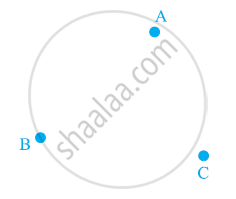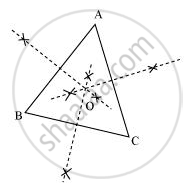Share

# In a huge park people are concentrated at three points (see the given figure): - Mathematics

Course

#### Question

In a huge park people are concentrated at three points (see the given figure):A: where there are different slides and swings for children,

B: near which a man-made lake is situated,

C: which is near to a large parking and exit.

Where should an ice-cream parlour be set up so that maximum number of persons can approach it?

(Hint: The parlor should be equidistant from A, B and C)

#### Solution

Maximum number of persons can approach the ice-cream parlour if it is equidistant from A, B and C. Now, A, B and C form a triangle. In a triangle, the circumcentre is the only point that is equidistant from its vertices. So, the ice-cream parlour should be set up at the circumcentre O of ΔABC.In this situation, maximum number of persons can approach it. We can find circumcentre O of this triangle by drawing perpendicular bisectors of the sides of this triangle.

Is there an error in this question or solution?